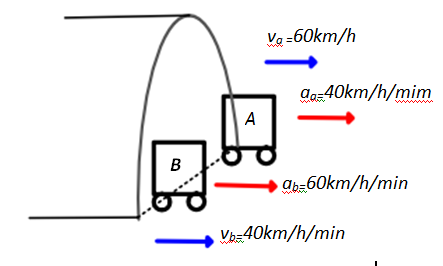×
Get Full Access to Physics: Principles With Applications - 6 Edition - Chapter 2 - Problem 2q
Get Full Access to Physics: Principles With Applications - 6 Edition - Chapter 2 - Problem 2q

×

# Can an object have varying speed if its velocity isISBN: 9780130606204 3

## Solution for problem 2Q Chapter 2

Physics: Principles with Applications | 6th Edition

• Textbook Solutions
• 2901 Step-by-step solutions solved by professors and subject experts
• Get 24/7 help from StudySoup virtual teaching assistantsPhysics: Principles with Applications | 6th Edition

4 5 1 286 Reviews
16
5
Problem 2Q

Problem 2Q

Can an object have varying speed if its velocity is constant? If yes, give examples.

Step-by-Step Solution:

Step 1 of 1The cars emerge from the tunnel at the same time. Since car A is faster than car B  at the same position, car A is passing car B. It will be in front of car B.

The car B has greater acceleration than A. If they continue with the same accelerations, after some time, the car B will have bigger velocity than A and catch and pass it.

Step 2 of 1

##### ISBN: 9780130606204

This full solution covers the following key subjects: constant, Examples, give, its, Object. This expansive textbook survival guide covers 35 chapters, and 3914 solutions. This textbook survival guide was created for the textbook: Physics: Principles with Applications, edition: 6. Since the solution to 2Q from 2 chapter was answered, more than 385 students have viewed the full step-by-step answer. The answer to “Can an object have varying speed if its velocity is constant? If yes, give examples.” is broken down into a number of easy to follow steps, and 15 words. Physics: Principles with Applications was written by and is associated to the ISBN: 9780130606204. The full step-by-step solution to problem: 2Q from chapter: 2 was answered by , our top Physics solution expert on 03/03/17, 03:53PM.

## Discover and learn what students are asking

Calculus: Early Transcendental Functions : Basic Differentiation Rules and Rates of Change
?Finding a Value In Exercises 65–70, find k such that the line is tangent to the graph of the function. Function

Chemistry: The Central Science : The Chemistry of Life: Organic and Biological Chemistry
?(a) Which of the following compounds, if any, is an ether? (b) Which compound, if any, is an alcohol? (c) Which compound, if any,

Unlock Textbook Solution

Enter your email below to unlock your verified solution to:

Can an object have varying speed if its velocity is